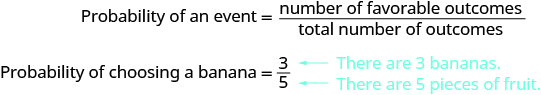## Finding the Probability of an Event

### Learning Outcomes

• Apply the basic definition of probability

The probability of an event tells us how likely that event is to occur. We usually write probabilities as fractions or decimals.
For example, picture a fruit bowl that contains five pieces of fruit – three bananas and two apples.

If you want to choose one piece of fruit to eat for a snack and don’t care what it is, there is a ${\Large\frac{3}{5}}$ probability you will choose a banana, because there are three bananas out of the total of five pieces of fruit. The probability of an event is the number of favorable outcomes divided by the total number of outcomes.### Probability

The probability of an event is the number of favorable outcomes divided by the total number of outcomes possible.

$\text{Probability}={\Large\frac{\text{number of favorable outcomes}}{\text{total number of outcomes}}}$

Converting the fraction ${\Large\frac{3}{5}}$ to a decimal, we would say there is a $0.6$ probability of choosing a banana.

$\begin{array}{}\\ \text{Probability of choosing a banana}={\Large\frac{3}{5}}\hfill \\ \text{Probability of choosing a banana}=0.6\hfill \end{array}$

This basic definition of probability assumes that all the outcomes are equally likely to occur. If you study probabilities in a later math class, you’ll learn about several other ways to calculate probabilities.

### example

The ski club is holding a raffle to raise money. They sold $100$ tickets. All of the tickets are placed in a jar. One ticket will be pulled out of the jar at random, and the winner will receive a prize. Cherie bought one raffle ticket.
1. Find the probability she will win the prize.
2. Convert the fraction to a decimal.

Solution

 1. What are you asked to find? The probability Cherie wins the prize. What is the number of favorable outcomes? $1$, because Cherie has $1$ ticket. Use the definition of probability. $\text{Probability of an event}={\Large\frac{\text{number of favorable outcomes}}{\text{total number of outcomes}}}$ Substitute into the numerator and denominator. $\text{Probability Cherie wins}={\Large\frac{1}{100}}$
 2. Convert the fraction to a decimal. Write the probability as a fraction. $\text{Probability}={\Large\frac{1}{100}}$ Convert the fraction to a decimal. $\text{Probability}=0.01$

### example

Three women and five men interviewed for a job. One of the candidates will be offered the job.
1. Find the probability the job is offered to a woman.
2. Convert the fraction to a decimal.

### TRY IT

The following video contains another example of how to compute the probability of an event and write it as either a fraction or decimal.

## Contribute!

Did you have an idea for improving this content? We’d love your input.